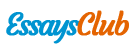# You Have Three European 3-Month Xyz Calls with Exercise Prices 100,120 and 130. the Calls Are at \$8, 5 and 3, Respectively. Do We See Any Arbitrage Opportunity?

Autor:   •  November 16, 2017  •  724 Words (3 Pages)  •  415 Views

Page 1 of 3

...

Also, Δ=δC/δS=N(d1). Equivalently,

ηC=SN(d1)/ [SN(d1)-Xe-rtN(d2)].

Since Xe-rtN(d2)>0, 0-rtN(d2)We can conclude that

ηC=SN(d1)/ [SN(d1)-Xe-rtN(d2)] ≥1.

Therefore, the percentage change in the option value is larger than the percentage change in the underlying asset price. A call option is riskier than the underlying asset.

- You have a stock trading at \$100. The stock follows a lognormal distribution with drift of 20% and volatility of 40%. The risk free rate is 3%.

What is the probability for a 4-month 100put to expire in the money? Find N(-d2). Compare the result.

The required probability is the probability of the stock price being less than \$100 in four month time. Suppose that the stock price in four months is St. Then,

lnSt ~ φ(ln100+(0.2-0.42/2)*1/3, 0.42*1/3)

i.e., lnSt ~ φ(4.645,0.2312)

Since ln 100=4.605, the required probability is N((4.605-4.645)/ 0.231)=N(-0.173).

From normal distribution tables, N(-0.173)=0.431. So that the probability for a 4-month 100put to expire in the money is 43.1%.

According to the BS Model, d1=[ln(100/100)+(0.03+0.42/2)*1/3]/ 0.4*√1/3=0.1588

d2=d1-0.4*√1/3= - 0.0722. Then, we can derive N(-d2) from normal distribution table: N(-d2)=0.5288.

In comparison, the actual probability (43.1%) is lower than N (-d2)=52.8%. Because the expected return on the stock exceeds the risk-free rate, the actual probability of the put expiring in the money will be lower than the risk-neutral probability.

What is the risk-neutral probability for a 4-month 100put to expire in the money? Find N(-d2). Compare the results.

In a risk-neutral world, lnSt ~ φ(lnS0+(R-σ 2/2)*T, σ 2*T), Where R is the risk-free rate. So our lnSt will be normally distributed with:

lnSt ~ φ(ln100+(0.03-0.42/2)*1/3, 0.42*1/3)

i.e., lnSt ~ φ(4.5885,0.2312)

Since ln100=4.605, the risk-neutral probability is N((4.605-4.5885)/0.231)= N(0.0714). From normal distribution tables, N(0.0714)=0.528. So that the risk-neutral probability for a 4-month 100put to expire in the money is 52.88%.

Obviously, the risk-neutral probability (52.8%) is equal to N(-d2)=52.8%. This is because the risk-neutral probability is taking the expected return on the stock to be the risk-free rate. Hence, N(-d2) works out to exactly the risk-neutral probability of the put expiring in the money.

...